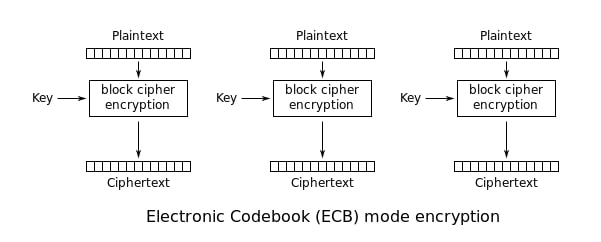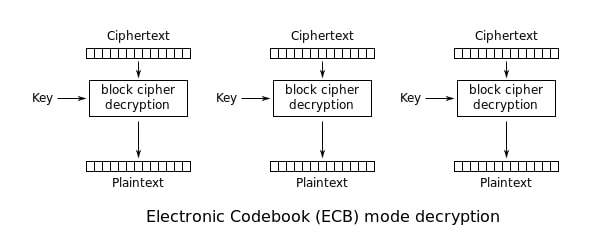# Simple Attack On AES-ECB Mode

## PicoCTF-18 (SpyFi ,300 pt)

In this challange they use AES-ECB mode which we know it is quite vulnerable.

If you dont know how AES-ECB works i would suggest to read about first. AES-ECB

AES-ECB Encryption and Decryption flow chartNow come to the challange

``````#!/usr/bin/python2 -u
from Crypto.Cipher import AES

agent_code = """flag"""

if len(message) % 16 != 0:
message = message + '0'*(16 - len(message)%16 )    #block-size = 16
return message

def encrypt(key, plain):
cipher = AES.new( key.decode('hex'), AES.MODE_ECB )
return cipher.encrypt(plain).encode('hex')

welcome = "Welcome, Agent 006!"
print welcome

message = '''Agent,
Greetings. My situation report is as follows:
{0}                                                     #here is our input message
My agent identifying code is: {1}.                      #flag
Down with the Soviets,
006'''.format(sitrep,agent_code)

print encrypt( """key""", message )
``````

lets send input message : `AAAAAAAAAAA + BBBBBBBBBBBBBBBB + CCCCCCCCCCCCCCCC <-- 'A'x11 (offset) + 'B'x16 + 'C'x16`

suppose flag is : `picoCTF{ABCDEFG}`

Divide message in blocks of 16

``````   'Agent,\nGreetings' <--- 16  (Block 1)
'. My situation r'  <--- 16  (Block 2)
'eport is as foll'  <--- 16  (Block 3)
'ows:\nAAAAAAAAAAA' <--- 16  (Block 4)
'BBBBBBBBBBBBBBBB'  <--- 16  (Block 5)
'CCCCCCCCCCCCCCCC'  <--- 16  (Block 6)
'\nMy agent identi' <--- 16  (Block 7)
'fying code is:  '  <--- 16  (Block 8)  <---known Block
'picoCTF{ABCDEFG}'  <--- 16  (Block 9)  <---unknown Block
'.Down with the S'  <--- 16  (Block 10) <---known Block
``````

Now if we send input with one less ‘C’ then blocks is:

``````   'Agent,\nGreetings'   <--- 16  (Block 1)
'. My situation r'    <--- 16  (Block 2)
'eport is as foll'    <--- 16  (Block 3)
'ows:\nAAAAAAAAAAA'   <--- 16  (Block 4)
'BBBBBBBBBBBBBBBB'    <--- 16  (Block 5)
'CCCCCCCCCCCCCCC\n'   <--- 16  (Block 6)
'My agent identif'    <--- 16  (Block 7)
'ying code is:  p'    <--- 16  (Block 8) <---here is our one byte flag but we dont know what char is it.
'icoCTF{ABCDEFG}.'    <--- 16  (Block 9) <---unknown block
'Down with the So'    <--- 16  (Block 10)<---known Block
``````

Now we know the encryption of block 8 in which last byte is our flag’s first byte, to know what byte is we send input like this

``````   'Agent,\nGreetings'    <--- 16  (Block 1)
'. My situation r'     <--- 16  (Block 2)
'eport is as foll'     <--- 16  (Block 3)
'ows:\nAAAAAAAAAAA'    <--- 16  (Block 4)
'ying code is:  %s'    <--- 16  (Block 5)    <--- replace %s to char in range(32,128)
'CCCCCCCCCCCCCCC\n'    <--- 16  (Block 6)
'My agent identif'     <--- 16  (Block 7)
'ying code is:  p'     <--- 16  (Block 8)    <--- Now we know block 8 has our one byte flag
'icoCTF{ABCDEFG}.'     <--- 16  (Block 9)    <--- unknown block
'Down with the So'     <--- 16  (Block 10)   <---known Block
``````

What we have to do is to check the encryption of block 5 and block 8 over the loop if it is same means the char we send is our first char of flag!! Whooho…!! we just get the 1st byte of unknown block without knowing key, lets repeat again same method with 2 ‘C’ less.

after 1st loop we know flag starting with : “p”

Now for second byte send input like this

``````   'Agent,\nGreetings'    <--- 16  (Block 1)
'. My situation r'     <--- 16  (Block 2)
'eport is as foll'     <--- 16  (Block 3)
'ows:\nAAAAAAAAAAA'    <--- 16  (Block 4)
'ing code is: p%s'     <--- 16  (Block 5)    <--- replace %s to char in range(32,128)
'CCCCCCCCCCCCCC\nM'    <--- 16  (Block 6)
'y agent identify'     <--- 16  (Block 7)
'ing code is:  pi'     <--- 16  (Block 8)    <--- Now we know block 8 has our two byte flag in which one we know i.e "p" and other we have to find out in second loop.
'coCTF{ABCDEFG}.D'     <--- 16  (Block 9)    <--- unknown block
'own with the Sov'     <--- 16  (Block 10)   <--- known Block
``````

Repeat process again check encryption of block 5 and block 8 if it same means the second char we send is our flag’s second char..!!

after 2nd loop we know flag starting with : “pi”

Same process we have to do until we get full flag!!!

My Script for this challenge

``````#!/usr/bin/python2 -u

from pwn import *
flag = "picoCTF{"

for j in range(1,14):
print(".................................................",j)
p = remote('2018shell1.picoctf.com', 37131)
my_msg = "A"*11+"B"*(25-j)
p.sendline(my_msg)
enc_msg = p.recv(1024).decode('hex')
p.close()

for i in range(32,128):
q = remote('2018shell1.picoctf.com', 37131)
msg = "A"*11+"B"*(14-j) + flag + chr(i)

q.sendline(msg)
y = q.recv(1024).decode('hex')
q.close()
if y[80:96] == enc_msg[128:144]:
flag += chr(i)
break
print(flag)

#picoCTF{@g3nt6_1\$_th3_c00l3\$t_2432504}

``````
Crypto, PicoCTF-18, SpyFi, AES-ECB